The CORR Procedure
 Partial Correlation

A partial correlation measures the strength of a relationship between two variables, while controlling the effect of other variables. The Pearson partial correlation between two variables, after controlling for variables in the PARTIAL statement, is equivalent to the Pearson correlation between the residuals of the two variables after regression on the controlling variables.

Let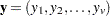be the set of variables to correlate and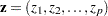be the set of controlling variables. The population Pearson partial correlation between theth and theth variables of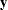givenis the correlation between errors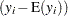and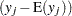, where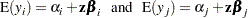are the regression models for variables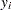and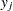given the set of controlling variables, respectively.

For a given sample of observations, a sample Pearson partial correlation betweenandgivenis derived from the residuals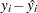and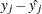, where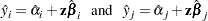are fitted values from regression models for variablesandgiven.

The partial corrected sums of squares and crossproducts (CSSCP) ofgivenare the corrected sums of squares and crossproducts of the residuals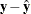. Using these partial corrected sums of squares and crossproducts, you can calculate the partial covariances and partial correlations.

PROC CORR derives the partial corrected sums of squares and crossproducts matrix by applying the Cholesky decomposition algorithm to the CSSCP matrix. For Pearson partial correlations, let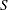be the partitioned CSSCP matrix between two sets of variables,and: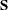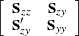PROC CORR calculates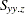, the partial CSSCP matrix ofafter controlling for, by applying the Cholesky decomposition algorithm sequentially on the rows associated with, the variables being partialled out.

After applying the Cholesky decomposition algorithm to each row associated with variables, PROC CORR checks all higher-numbered diagonal elements associated withfor singularity. A variable is considered singular if the value of the corresponding diagonal element is less thantimes the original unpartialled corrected sum of squares of that variable. You can specify the singularity criterionby using the SINGULAR= option. For Pearson partial correlations, a controlling variableis considered singular if thefor predicting this variable from the variables that are already partialled out exceeds
. When this happens, PROC CORR excludes the variable from the analysis. Similarly, a variable is considered singular if thefor predicting this variable from the controlling variables exceeds. When this happens, its associated diagonal element and all higher-numbered elements in this row or column are set to zero.

After the Cholesky decomposition algorithm is applied to all rows associated with, the resulting matrix has the form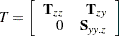where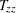is an upper triangular matrix with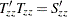,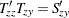, and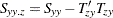.

If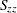is positive definite, then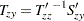and the partial CSSCP matrixis identical to the matrix derived from the formula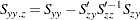The partial variance-covariance matrix is calculated with the variance divisor (VARDEF= option). PROC CORR then uses the standard Pearson correlation formula on the partial variance-covariance matrix to calculate the Pearson partial correlation matrix.

When a correlation matrix is positive definite, the resulting partial correlation between variables x and y after adjusting for a single variable z is identical to that obtained from the first-order partial correlation formula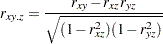where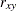,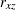, and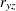are the appropriate correlations.

The formula for higher-order partial correlations is a straightforward extension of the preceding first-order formula. For example, when the correlation matrix is positive definite, the partial correlation between x and y controlling for both z_1 and z_2 is identical to the second-order partial correlation formula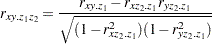where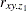,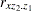, and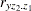are first-order partial correlations among variables x, y, and z_2 given z_1.

To derive the corresponding Spearman partial rank-order correlations and Kendall partial tau-b correlations, PROC CORR applies the Cholesky decomposition algorithm to the Spearman rank-order correlation matrix and Kendall’s tau-b correlation matrix and uses the correlation formula. That is, the Spearman partial correlation is equivalent to the Pearson correlation between the residuals of the linear regression of the ranks of the two variables on the ranks of the partialled variables. Thus, if a PARTIAL statement is specified with the CORR=SPEARMAN option, the residuals of the ranks of the two variables are displayed in the plot. The partial tau-b correlations range from –1 to 1. However, the sampling distribution of this partial tau-b is unknown; therefore, the probability values are not available.

### Probability Values

Probability values for the Pearson and Spearman partial correlations are computed by treating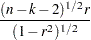as coming from a t distribution with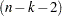degrees of freedom, whereis the partial correlation andis the number of variables being partialled out.Previous Page | Next Page | Top of Page